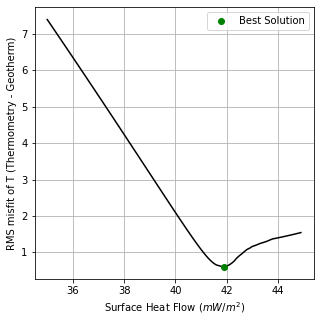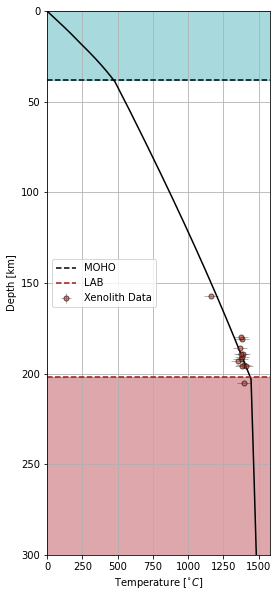This page was generated from docs/Examples/Garnet_Geotherms/Geotherm_functions.ipynb. Interactive online version:.

# Garnet Geotherms

[ ]:

import numpy as np
import matplotlib.pyplot as plt
import Thermobar as pt

:

#Here let's recalculate T-P from the study of Tappe et al. (2021).
#First creating the composition arrays.
out=pt.import_excel('Tappe2021.xlsx', sheet_name="Sheet1")
myOpxs = out['Opxs']
myCpxs = out['Cpxs']
myGts = out['Gts']
#Iterative solution of Brey and Köhler (1990) for the given dataset.
calc=pt.calculate_pyroxenes_garnet_press_temp(opx_comps=myOpxs, cpx_comps=myCpxs,
gt_comps = myGts, equationP="P_Brey1990",
equationT="T_Brey1990",T_K_guess = 1300)
calc

/home/sinan/.local/lib/python3.10/site-packages/pandas/core/indexing.py:2115: FutureWarning: In a future version, the Index constructor will not infer numeric dtypes when passed object-dtype sequences (matching Series behavior)
new_ix = Index(new_ix)
/home/sinan/.local/lib/python3.10/site-packages/pandas/core/indexing.py:2115: FutureWarning: In a future version, the Index constructor will not infer numeric dtypes when passed object-dtype sequences (matching Series behavior)
new_ix = Index(new_ix)

:

P_kbar_calc T_K_calc
0 62.186165 1665.508418
1 59.185112 1638.988377
2 60.174362 1663.121565
3 61.142703 1644.163358
4 62.367269 1680.010277
5 60.658036 1653.928696
6 60.041928 1648.787123
7 62.232799 1654.135943
8 65.247289 1667.306600
9 57.612930 1653.004617
10 61.387758 1627.492544
11 49.661312 1435.644159
12 57.120652 1646.498971
:

T_ext = calc['T_K_calc']
P_ext = calc['P_kbar_calc'] / 10.0

#Solving for SHF values between 35 (SHF_start) and 45 (SHF_end) for the function with increments of 0.1 (SHF increment)
#to minimise the RMS misfit function find the best fitting geotherm.
#Kinked=True adds kinked geotherm at the temperature BDL_T, parallel to the D-G transition.
#max_depth=maximum depth until the geotherm is calculated.
#plot_solution=True returns a graph of minimisation process.
shf_solution, T_solution, depth_solution, p_solution, misfit_solution = pt.invert_generalised_mantle_geotherm(P_sample = P_ext, T_sample = T_ext, std_P = 0.3, std_T = 50,
SHF_start = 35, SHF_end=45, SHF_increment=0.1, max_depth=300, kinked=False, BDL_T = 170, adiabat = True,
plot_solution = True):

#Making a plot of the solution.
pt.mantle_geotherm_plot(T = T_solution, P = p_solution, Depth = depth_solution,
plot_style = 'Depth', Temp_unit = 'Celsius',
T_Sample = T_ext, P_Sample = P_ext, T_std = 50,
P_std = 0.3, plot_type = 'show', max_depth = 250,
moho = 38, lab = 202, leg = True):

help(pt.mantle_geotherm_plot)

Help on function mantle_geotherm_plot in module Thermobar.plotting:

mantle_geotherm_plot(T, P, Depth, plot_style, Temp_unit, T_Sample, P_Sample, T_std, P_std, max_depth, plot_type, **kwargs)
A function to plot calculate geotherm alongside the thermobarometric
calculations.

###Parameters###
T: Temperature array of the geotherm.

P: Pressure array of the geotherm.

Depth: Depth array of the geotherm in meters.

plot_style: String parameter for the y-axis of the geotherm plot 'Pressure' or 'Depth'.

Temp_unit: String parameter for the temperature unit, 'Celsius' or 'Kelvin'.

T_Sample: Array of temperature of the thermobarometric solutions.

P_Sample: Array of pressure of the thermobarometric solutions in GPa.

T_std: Standart deviation of thermobarometric temperature estimation. Could be array or a single value.

P_std: Standart deviation of thermobarometric pressure estimation. Could be array or a single value.

max_depth: Maximum depth to show the plot.

leg: Boolean parameter to set existence of a legend.

plot_type: 'show' or 'save' the figure.

moho: moho depth in km.

lab: lab depth in km.

Depth_Sample: Array of depths of the thermobarometric solutions.

filename_save: string parameter for filename to save the figures.


[ ]: